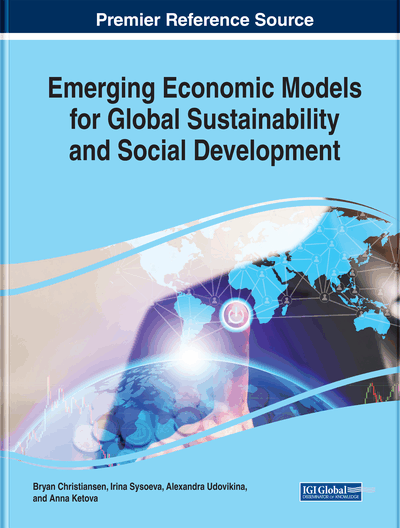# The Modelling of the Economy by Means of C-V-M Matrices

Grigorii S. Pushnoi
DOI: 10.4018/978-1-5225-5787-6.ch018
OnDemand:
(Individual Chapters)
Available
\$29.50
No Current Special Offers

## Abstract

The classical three-sector model of the economy: 1) “the means of production”, 2) “the goods for employees”, and 3) “the goods consumed by other economic agents” (“luxury goods”) is considered in matrix formulation. Each sector contains many industries producing the goods of these three kinds. The “transformation problem” in Marxian economics is considered in a three-sector model of the economy with simple production. The solution of this problem is based on the action of the statistical “laws of large numbers” (LLN) in the economy. The stylized facts about the economy of the United States indicate onto the existence of the following probability distributions: 1) the inverse power distribution for the elements of matrix of direct requirements and 2) the Gaussian distribution for the direct labor per the unit of goods. The action of the statistic “law of large numbers” guarantees the C-V-M matrix of the economy must be almost symmetric. The “labor value” and the “price of production” of the total product produced within each sector in this case are almost equal.
Chapter Preview
Top

## Introduction

The Three-sector model of the economy was first formulated in the second volume of “Capital” (Marx, 1885; ch.20). Later many economists, beginning with Tugan-Baranovsky (1906) and Bortkiewicz (1907), used this model for consideration of the “problem of transformation” of “labor values” into “prices of production”. Marx has formulated the following two rules of this transformation in the third volume of the “Capital” (Marx, 1894; ch.9):

• The First Rule of Transformation: The aggregate profit in the economy accumulated for example during the year equals the aggregate surplus value created by the labor of employees;

• The Second Rule of Transformation: The “value” (embodied labor in the money form) of the aggregate product produced during the year equals to its price of production. Bortkiewicz (1907a; 1907b) in 1907 has considered the transformation of the “labor values” into the “prices of production” using the three-sector model of the economy with simple production. He discovered that Marx’s transformational rules could not be performed simultaneously in the economy with arbitrary structure. Only one rule of transformation can be performed in the general case: either the sum of the profits equals to the aggregate surplus value or the price and the value of aggregate output are equal. Bortkiewicz concluded about logical inconsistence in Marx’s theory. Therefore, the “transformation problem” was created.

This discouraging result shattered confidence in the Marx’s “labor theory of value”. Later many scientists came to the same conclusion (Sweezy, 1949; Meek, 1956; Samuelson 1957, 1971; Medio, 1972; Steedman, 1977; Abraham-Frois (1979); Itoh, 1980) and now a majority of economists are convinced that Marx’s theory is internally inconsistent. Numerous attempts have been undertaken to solve this problem. Morishima and Caterhores (1975) have assumed the Marxian algorithm of transformation is only the first step of the iterative process. Shaikh (1977, 1984) has assumed that the solution of this problem can be obtained as a result of sequence of iterations of the Marx’s transformation algorithm.

Sweezy (1949) has assumed that the problem can be solved if, in reality, “values” and “prices of production” are connected by a nonlinear relation. Lipietz (1982), Dumenil (1980, 1983), and Foley (1982) have offered a so-called “new interpretation” of “transformational rules” – the so-called “new solution”. Freeman (1996), Kliman and McGlone (1999), and Kliman (2007) have offered “temporal single-system interpretation” (TSSI) in which “output prices” and “input prices” can differ in each period because the real economy is not the equilibrium dynamical system. Kalyuzhnyi (2014) proposed solution based on nontrivial interpretation of “spheres of production” as complexes of industries producing only the goods of final consumption.

## Complete Chapter List

Search this Book:
Reset